Question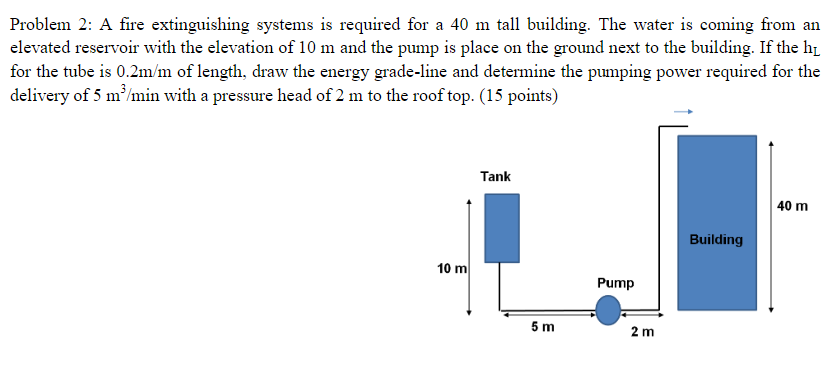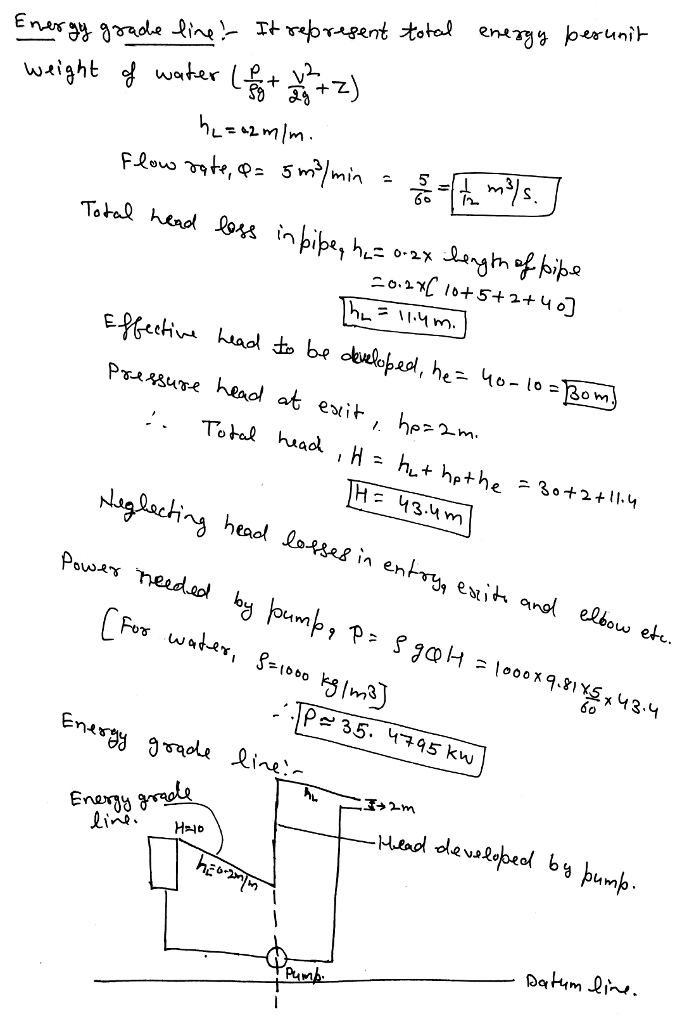#### Earn Coins

Coins can be redeemed for fabulous gifts.

Similar Homework Help Questions
• ### Problemi 2: A man stands on the roof of a 20.0 m tall building and throws...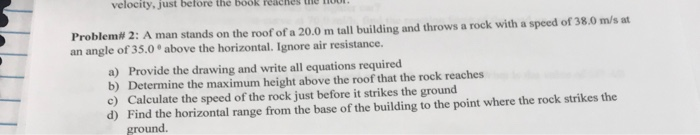Problemi 2: A man stands on the roof of a 20.0 m tall building and throws a rock with a speed of 38.0 m/s an angle of 35.0 above the horizontal. Ignore air resistance. a) Provide the drawing and write all equations required b) Determine the maximum height above the roof that the rock reaches c) Calculate the speed of the rock just before it strikes the ground d) Find the horizontal range from the base of the building to...

• ### Task 1: You were asked to check up the status of the water system. Water at 24 °C is to be pumped from a Lower reservoir with 100 m elevation to another reservoir at a higher elevation 112 m through...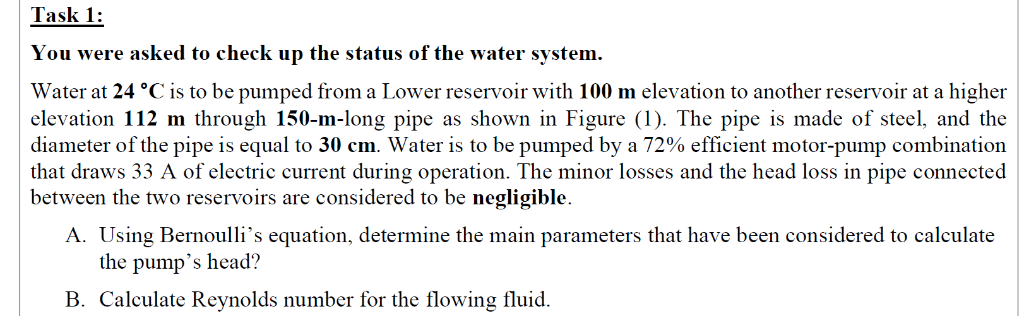Task 1: You were asked to check up the status of the water system. Water at 24 °C is to be pumped from a Lower reservoir with 100 m elevation to another reservoir at a higher elevation 112 m through 150-m-long pipe as shown in Figure (1). The pipe is made of steel, and the diameter of the pipe is equal to 30 cm. Water is to be pumped by a 72% efficient motor-pump combination that draws 33 A of...

• ### 3. (20%) Water of 15°C temperature is pumped at a rate of 0.01 m/s through the...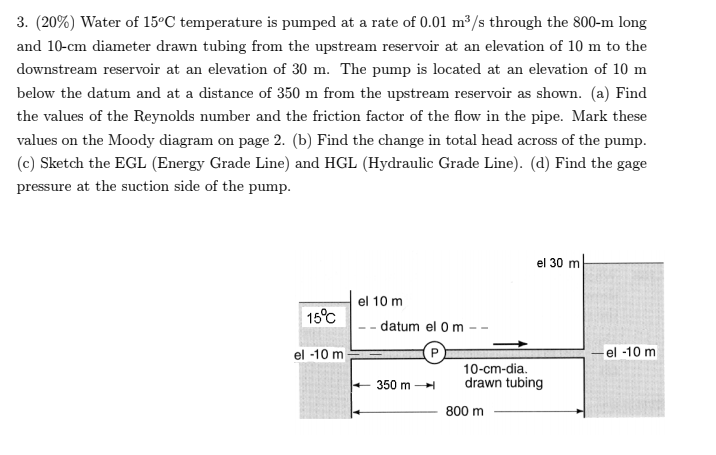3. (20%) Water of 15°C temperature is pumped at a rate of 0.01 m/s through the 800-m long and 10-cm diameter drawn tubing from the upstream reservoir at an elevation of 10 m to the downstream reservoir at an elevation of 30 m. The pump is located at an elevation of 10 m below the datum and at a distance of 350 m from the upstream reservoir as shown. (a) Find the values of the Reynolds number and the friction...

• ### Problem 1: In the reservoir-pipe system below, water is supplied from reservoir A to reservoir B...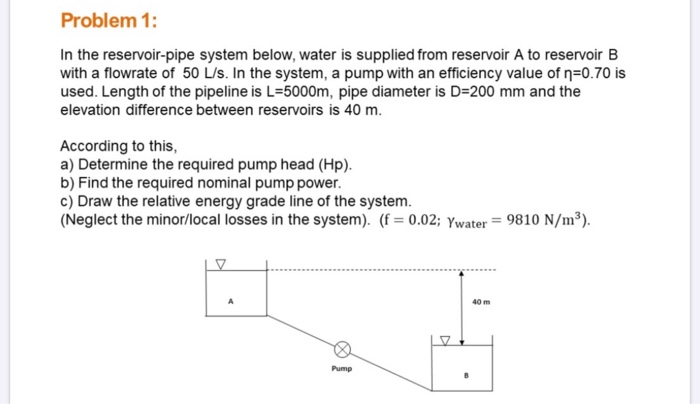Problem 1: In the reservoir-pipe system below, water is supplied from reservoir A to reservoir B with a flowrate of 50 L/S. In the system, a pump with an efficiency value of n=0.70 is used. Length of the pipeline is L=5000m, pipe diameter is D=200 mm and the elevation difference between reservoirs is 40 m. According to this, a) Determine the required pump head (Hp). b) Find the required nominal pump power. c) Draw the relative energy grade line of...

• ### you can skip task 1 (3) because its a code in matlab. Task 1: You were asked to check up the status of the water system. Water at 24 °C is to be pumped from a Lower reservoir with 100 m elevation to...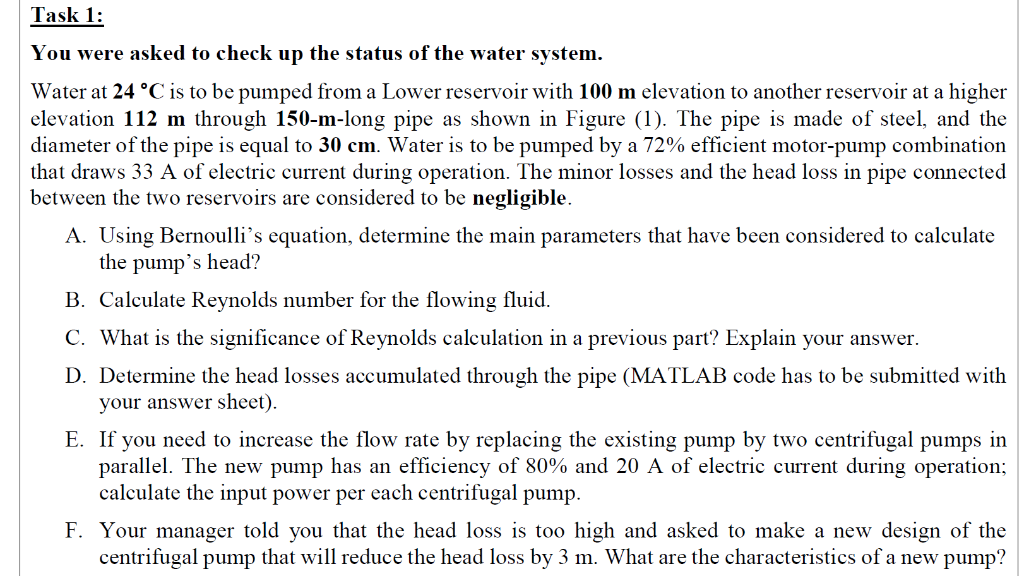you can skip task 1 (3) because its a code in matlab. Task 1: You were asked to check up the status of the water system. Water at 24 °C is to be pumped from a Lower reservoir with 100 m elevation to another reservoir at a higher elevation 112 m through 150-m-long pipe as shown in Figure (1). The pipe is made of steel, and the diameter ofthe pipe is equal to 30 cm water is to be pumped...

• ### water resources (4) A pump is required to deliver 150 L/s through a 300-mm diameter PVC...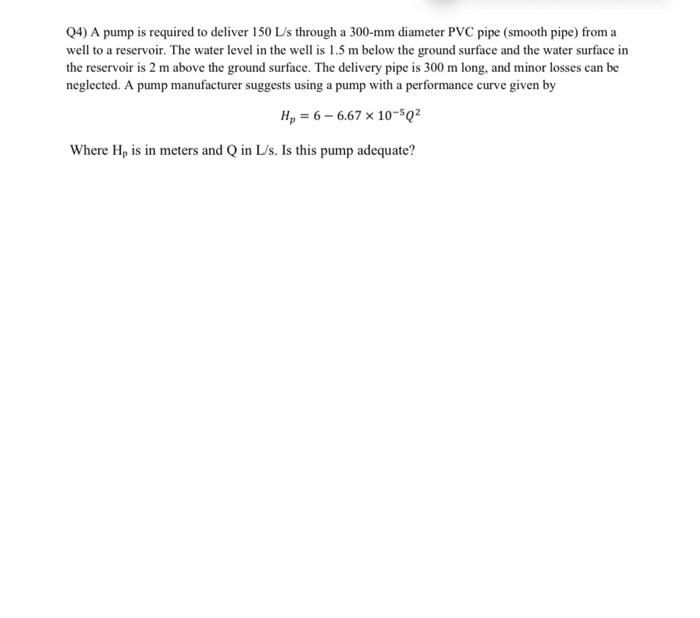water resources (4) A pump is required to deliver 150 L/s through a 300-mm diameter PVC pipe (smooth pipe) from a well to a reservoir. The water level in the well is 1.5 m below the ground surface and the water surface in the reservoir is 2 m above the ground surface. The delivery pipe is 300 m long, and minor losses can be neglected. A pump manufacturer suggests using a pump with a performance curve given by | HA...

• ### The performance data of a water pump follow the curve fit H available = H0 - aV˙2Havailable = H0 - aV˙2 where the pump’s...

The performance data of a water pump follow the curve fit H available = H0 - aV˙2Havailable = H0 - aV˙2 where the pump’s shutoff head H0 = 7.6 m, coefficient a = 0.0453 m/(Lpm)2, the units of pump head H are meters, and the units of  V˙V˙ are liters per minute (Lpm). The pump is used to pump water from one large reservoir to another large reservoir at a higher elevation. The free surfaces of both reservoirs are exposed...

• ### Problem 10 7.50 Water (10°C) is flowing at a rate of 0.35 m/s, and it is...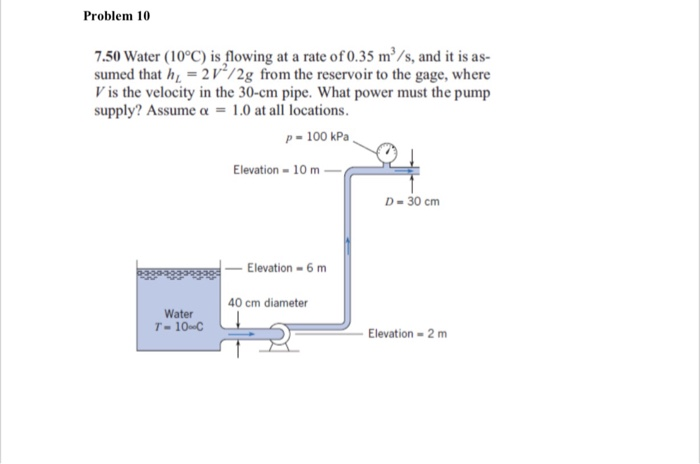Problem 10 7.50 Water (10°C) is flowing at a rate of 0.35 m/s, and it is as- sumed that h1 = 2 V"/2g from the reservoir to the gage, where V is the velocity in the 30-cm pipe. What power must the pump supply? Assume a-1.0 at all locations P-100 kPa Elevation10m D-30 cm 3853939333Elevation 6 m 40 cm diameter Water T-10-c Elevation-2m

• ### Remaining Time: 25 minutes, 42 seconds. Question Completion Status: QUESTION 6 22 The pump system in...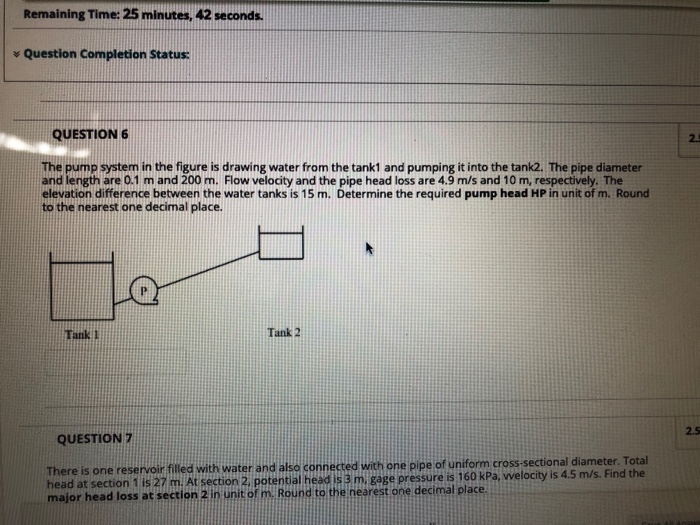Remaining Time: 25 minutes, 42 seconds. Question Completion Status: QUESTION 6 22 The pump system in the figure is drawing water from the tank1 and pumping it into the tank2. The pipe diameter and length are 0.1 m and 200 m. Flow velocity and the pipe head loss are 4.9 m/s and 10 m, respectively. The elevation difference between the water tanks is 15 m. Determine the required pump head HP in unit of m. Round to the nearest one...

• ### 10 peles A 3-m-diameter tank is initially filled with water 2 m above the center of...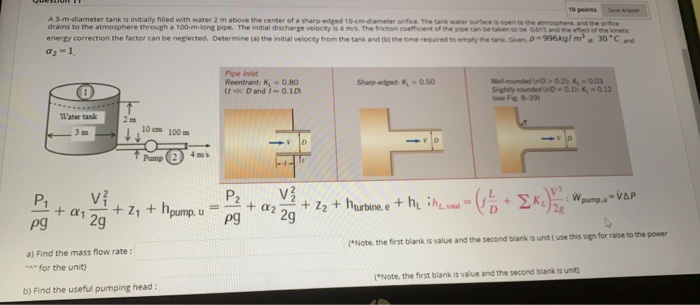10 peles A 3-m-diameter tank is initially filled with water 2 m above the center of a sharp-edged 10 cm diameter orifice. The tank water surface is open to the other, and the orice drains to the atmosphere through a 100-m-long pipe. The initial discharge velocity is 4 m/s. The friction coefficient of the can be when to be 0.015 and the effect of the energy correction the factor can be neglected. Determine (a) the initial velocity from the tank...